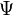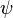# Psi (letter) facts for kids

Kids Encyclopedia Facts
 Quick facts for kidsGreek alphabet Other letters Αα Alpha Νν Nu Ββ Beta Ξξ Xi Γγ Gamma Οο Omicron Δδ Delta Ππ Pi Εε Epsilon Ρρ Rho Ζζ Zeta Σσς Sigma Ηη Eta Ττ Tau Θθ Theta Υυ Upsilon Ιι Iota Φφ Phi Κκ Kappa Χχ Chi Λλ Lambda Ψψ Psi Μμ Mu Ωω Omega Ϝϝ Digamma Ⱶⱶ Heta Ϻϻ San Ϙϙ Koppa Ϡϡ Sampi Ϛϛ Stigma Ϸϸ Sho

Psi (uppercase/lowercase Ψ ψ) is the 23rd letter of the Greek alphabet. It is used to represent the "ps" sound in Ancient and Modern Greek. In the system of Greek numerals, it has a value of 700. Letters that came from it include Cyrillic Ѱ.

In mathematics, the uppercase$\Psi$ is used as a variable for sets of sentences (in logic), and the lowercase$\psi$ is used to represent the sum of reciprocals of Fibonacci numbers. Other applications include the polygamma function, the gamma function and the super golden ratio.

## Images for kidsPsi (letter) Facts for Kids. Kiddle Encyclopedia.